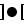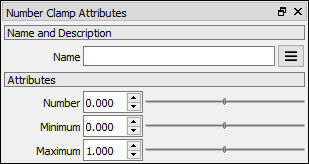# Number Clamp node

Left Toolbar >Math Nodes >Number Clamp

The Number Clamp node returns the nearest integer to the Number value in the defined area.

## Procedure

### To calculate the clamp of the number in the node graph:

1. On the left toolbar choose Math Nodes> Number Clamp2. Drag the Number Clamp icon into the Node Graph.3. Connect the Output: result.

## Inputs

number A NUMBER type of value.

## Outputs

Result The number value.

## Attributes PanelNumber Number value. This attribute is exposed as input by default.

Minimum Minimum Number value of the range. This attribute is exposed as input by default.

Maximum Maximum Number value of the range. This attribute is exposed as input by default.

Math Nodes

Main Page